#### Simplicial Complex

For this definition, it is assumed that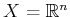. Let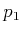,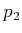,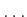,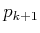, be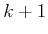linearly independent6.5 points in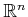. A-simplex,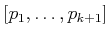, is formed from these points as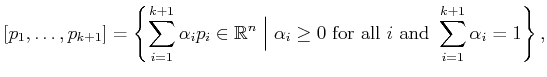(6.3)

in which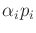is the scalar multiplication of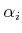by each of the point coordinates. Another way to view (6.3) is as the convex hull of thepoints (i.e., all ways to linearly interpolate between them). If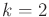, a triangular region is obtained. For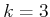, a tetrahedron is produced.

For any-simplex and anysuch that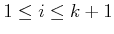, let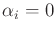. This yields a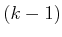-dimensional simplex that is called a face of the original simplex. A 2-simplex has three faces, each of which is a 1-simplex that may be called an edge. Each 1-simplex (or edge) has two faces, which are 0-simplexes called vertices.

To form a complex, the simplexes must fit together in a nice way. This yields a high-dimensional notion of a triangulation, which in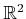is a tiling composed of triangular regions. A simplicial complex,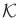, is a finite set of simplexes that satisfies the following:

1. Any face of a simplex inis also in.
2. The intersection of any two simplexes inis either a common face of both of them or the intersection is empty.
Figure 6.15 illustrates these requirements. For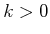, a-cell ofis defined to be interior,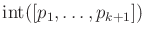, of any-simplex. For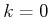, every 0-simplex is a 0-cell. The union of all of the cells forms a partition of the point set covered by. This therefore provides a cell decomposition in a sense that is consistent with Section 6.2.2.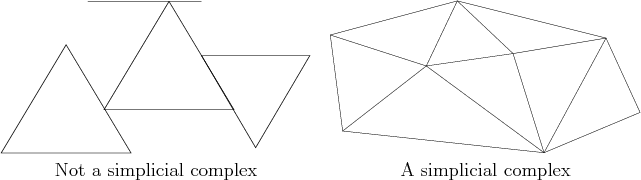Steven M LaValle 2020-08-14